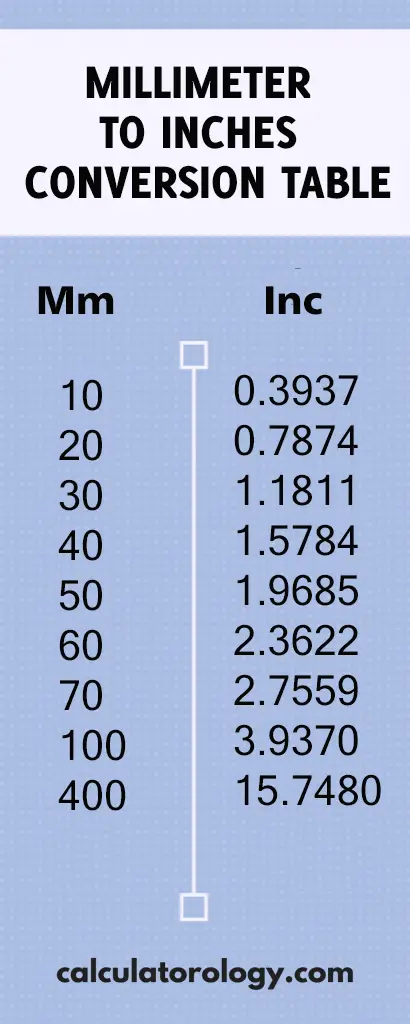## 2.7559055118 inches

### 70 mm to inches

The Millimeters to Inches conversion calculator is used to convert millimeters (mm) to inches (in). To convert 70 millimeters to inches, it is important to determine the number of inches that are equivalent to one millimeter.

#### How many inches in one millimeter?

1 millimeter = 0.03937007874 Inch

This means that there are 0.03937007874 inches in one millimeter. It can also be converted into decimals and shown as;

1 mm = (1/25.4) = 0.03937007874 inches.

D (“) = D (mm)/25.4

Therefore, to determine the distance in inches, you will divide the distance in millimeters by 25.4.## 70 mm to Inches Conversion

 mm inches

#### How to convert 70mm to inches

When using the converter, the first procedure is to enter the value 70 in the blank text field and then click the ‘Convert’ button to initiate the conversion.  It gives you quick and accurate results based on what you enter in the blank text field.

Since 1 millimeter is equivalent to 0.03937007874 inches, how many inches are there in 70 millimeters?

If 1 mm = 0.03937007874 inches,

70mm =? Inches

= (70 x 0.03937007874) inches

=2.7559055118 inches.

This implies that there are 2.7559055118 inches in 70 millimeters.

.You can still use the conversion calculator to perform more calculations using different values. The ‘Reset’ button is used to erase all information of the previous calculations to create a new platform for other calculations. The millimeter to inches conversion calculator can only execute a single conversion at a time.

70 mm to inches can also be determined using the Millimeter to Inches conversion table. It gives the conversion results of millimeters to inches based on a range of 0.01mm to 100mm.

##### Definitions

Millimeter is a length unit in metric measurements, abbreviated as “mm”. It is often used in measuring small distances in the fields of machining or engineering.

Inches

It is a US customary and imperial measurement system that is used widely and is abbreviated as (in) or using the symbol (“) - double prime.